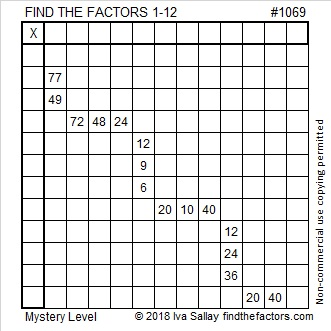# 1069 Mystery Level Puzzle

It isn’t a mystery where the 7’s and the 11’s go in this puzzle, but then what are you going to do? That’s the mystery. Can you write each number from 1 to 12 in both the first column and the top row so that those numbers are the factors of the given clues? There are plenty of places to be tricked in this puzzle, so good luck!Print the puzzles or type the solution in this excel file: 12 factors 1063-1072

Now I’ll write a little bit about the number 1069:

• 1069 is a prime number.
• Prime factorization: 1069 is prime.
• The exponent of prime number 1069 is 1. Adding 1 to that exponent we get (1 + 1) = 2. Therefore 1069 has exactly 2 factors.
• Factors of 1069: 1, 1069
• Factor pairs: 1069 = 1 × 1069
• 1069 has no square factors that allow its square root to be simplified. √1069 ≈ 32.695565

How do we know that 1069 is a prime number? If 1069 were not a prime number, then it would be divisible by at least one prime number less than or equal to √1069 ≈ 32.7. Since 1069 cannot be divided evenly by 2, 3, 5, 7, 11, 13, 17, 19, 23, 29 or 31, we know that 1069 is a prime number.The next prime number won’t be until 1087, making 1069 have a prime gap of 18. That’s two numbers short of the record for primes up to 1087, so it’s quite a lot!

Not only is 1069 a prime number, but OEIS.org informs us that
310693 is a prime number,
33106933 is a prime number,
3331069333 is a prime number, and
333310693333 is also a prime number!

30² + 13² = 1069

1069 is the hypotenuse of a Pythagorean triple:
731-780-1069 calculated from 30² – 13², 2(30)(13), 30² + 13²

Here’s another way we know that 1069 is a prime number: Since its last two digits divided by 4 leave a remainder of 1, and 30² + 13² = 1069 with 30 and 13 having no common prime factors, 1069 will be prime unless it is divisible by a prime number Pythagorean triple hypotenuse less than or equal to √1069 ≈ 32.7. Since 1069 is not divisible by 5, 13, 17, or 29, we know that 1069 is a prime number.

1069 looks interesting when it is written in a few other bases:
It’s palindrome 565 in BASE 14 because 5(14²) + 6(14) + 5(1) = 1069, and
4B4 in BASE 15 (B is 11 base 10) because 4(15²) + 11(15) + 4(1) = 1069, and
it’s consecutive odd digits 357 in BASE 18 because 3(18²) + 5(18) + 7(1) = 1069

This site uses Akismet to reduce spam. Learn how your comment data is processed.